• 本文原始地址：最大似然估计（Maximum likelihood estimation, 简称MLE）和最大后验概率估计（Maximum a posteriori estimation, 简称MAP）是很常用的两种参数估计方法，如果不理解这两种方法的思路，很
声明：本文为原创文章，发表于nebulaf91的csdn博客。欢迎转载，但请务必保留本信息，注明文章出处。
本文作者: nebulaf91
本文原始地址：http://blog.csdn.net/u011508640/article/details/72815981
最大似然估计（Maximum likelihood estimation, 简称MLE）和最大后验概率估计（Maximum a posteriori estimation, 简称MAP）是很常用的两种参数估计方法，如果不理解这两种方法的思路，很容易弄混它们。下文将详细说明MLE和MAP的思路与区别。
但别急，我们先从概率和统计的区别讲起。
概率和统计是一个东西吗？
概率（probabilty）和统计（statistics）看似两个相近的概念，其实研究的问题刚好相反。
概率研究的问题是，已知一个模型和参数，怎么去预测这个模型产生的结果的特性（例如均值，方差，协方差等等）。 举个例子，我想研究怎么养猪（模型是猪），我选好了想养的品种、喂养方式、猪棚的设计等等（选择参数），我想知道我养出来的猪大概能有多肥，肉质怎么样（预测结果）。
统计研究的问题则相反。统计是，有一堆数据，要利用这堆数据去预测模型和参数。仍以猪为例。现在我买到了一堆肉，通过观察和判断，我确定这是猪肉（这就确定了模型。在实际研究中，也是通过观察数据推测模型是／像高斯分布的、指数分布的、拉普拉斯分布的等等），然后，可以进一步研究，判定这猪的品种、这是圈养猪还是跑山猪还是网易猪，等等（推测模型参数）。
一句话总结：概率是已知模型和参数，推数据。统计是已知数据，推模型和参数。
显然，本文解释的MLE和MAP都是统计领域的问题。它们都是用来推测参数的方法。为什么会存在着两种不同方法呢？ 这需要理解贝叶斯思想。我们来看看贝叶斯公式。
贝叶斯公式到底在说什么？
学习机器学习和模式识别的人一定都听过贝叶斯公式(Bayes’ Theorem)：
P(A∣B)=P(B∣A)P(A)P(B)P(A|B) = \frac{P(B|A)P(A)}{P(B)} 【式1】
贝叶斯公式看起来很简单，无非是倒了倒条件概率和联合概率的公式。
把B展开，可以写成：
P(A∣B)=P(B∣A)P(A)P(B∣A)P(A)+P(B∣∼A)P(∼A)P(A|B) = \frac{P(B|A)P(A)}{P(B|A)P(A) + P(B|\sim A)P(\sim A)} 【式2】（∼A\sim A表示"非A"）
这个式子就很有意思了。
想想这个情况。一辆汽车（或者电瓶车）的警报响了，你通常是什么反应？有小偷？撞车了？  不。。 你通常什么反应都没有。因为汽车警报响一响实在是太正常了！每天都要发生好多次。本来，汽车警报设置的功能是，出现了异常情况，需要人关注。然而，由于虚警实在是太多，人们渐渐不相信警报的功能了。
贝叶斯公式就是在描述，你有多大把握能相信一件证据？（how much you can trust the evidence）
我们假设响警报的目的就是想说汽车被砸了。把A计作“汽车被砸了”，B计作“警报响了”，带进贝叶斯公式里看。我们想求等式左边发生A∣BA|B的概率，这是在说警报响了，汽车也确实被砸了。汽车被砸**引起（trigger）**警报响，即B∣AB|A。但是，也有可能是汽车被小孩子皮球踢了一下、被行人碰了一下等其他原因（统统计作∼A\sim A），其他原因引起汽车警报响了，即B∣∼AB|\sim A。那么，现在突然听见警报响了，这时汽车已经被砸了的概率是多少呢（这即是说，警报响这个证据有了，多大把握能相信它确实是在报警说汽车被砸了）？想一想，应当这样来计算。用警报响起、汽车也被砸了这事件的数量，除以响警报事件的数量（这即【式1】）。进一步展开，即警报响起、汽车也被砸了的事件的数量，除以警报响起、汽车被砸了的事件数量加上警报响起、汽车没被砸的事件数量（这即【式2】）。
可能有点绕，请稍稍想一想。
再思考【式2】。想让P(A∣B)=1P(A|B) = 1，即警报响了，汽车一定被砸了，该怎么做呢？让$P(B|\sim A)P(\sim A) = 0即可。很容易想清楚，假若让即可。很容易想清楚，假若让P(\sim A) = 0$，即杜绝了汽车被球踢、被行人碰到等等其他所有情况，那自然，警报响了，只剩下一种可能——汽车被砸了。这即是提高了响警报这个证据的说服力。
从这个角度总结贝叶斯公式：做判断的时候，要考虑所有的因素。 老板骂你，不一定是你把什么工作搞砸了，可能只是他今天出门前和太太吵了一架。
再思考【式2】。观察【式2】右边的分子，P(B∣A)P(B|A)为汽车被砸后响警报的概率。姑且仍为这是1吧。但是，若P(A)P(A)很小，即汽车被砸的概率本身就很小，则P(B∣A)P(A)P(B|A)P(A)仍然很小，即【式2】右边分子仍然很小，$P(A|B)$ 还是大不起来。 这里，​P(A)P(A)即是常说的先验概率，如果A的先验概率很小，就算P(B∣A)P(B|A)较大，可能A的后验概率P(A∣B)P(A|B)还是不会大（假设P(B∣∼A)P(∼A)P(B|\sim A)P(\sim A)不变的情况下）。
从这个角度思考贝叶斯公式：一个本来就难以发生的事情，就算出现某个证据和他强烈相关，也要谨慎。证据很可能来自别的虽然不是很相关，但发生概率较高的事情。 发现刚才写的代码编译报错，可是我今天状态特别好，这语言我也很熟悉，犯错的概率很低。因此觉得是编译器出错了。 ————别，还是先再检查下自己的代码吧。
好了好了，说了这么多，下面言归正传，说一说MLE。
——————不行，还得先说似然函数（likelihood function）
似然函数
似然（likelihood）这个词其实和概率（probability）是差不多的意思，Colins字典这么解释：The likelihood of something happening is how likely it is to happen. 你把likelihood换成probability，这解释也读得通。但是在统计里面，似然函数和概率函数却是两个不同的概念（其实也很相近就是了）。
对于这个函数：
P(x∣θ)P(x|\theta)
输入有两个：x表示某一个具体的数据；θ\theta表示模型的参数。
如果θ\theta是已知确定的，xx是变量，这个函数叫做概率函数(probability function)，它描述对于不同的样本点x，其出现概率是多少。
如果xx是已知确定的，θ\theta是变量，这个函数叫做似然函数(likelihood function), 它描述对于不同的模型参数，出现x这个样本点的概率是多少。
这有点像“一菜两吃”的意思。其实这样的形式我们以前也不是没遇到过。例如，$f(x, y) = x^y ,即, 即x的的y次方。如果次方。如果x是已知确定的(例如是已知确定的(例如x = 2)，这就是)，这就是f(y) = 2^y,这是指数函数。如果, 这是指数函数。 如果y是已知确定的(例如是已知确定的(例如y = 2)，这就是)，这就是f(x) = x^2$，这是二次函数。同一个数学形式，从不同的变量角度观察，可以有不同的名字。
这么说应该清楚了吧？ 如果还没讲清楚，别急，下文会有具体例子。
现在真要先讲讲MLE了。。
最大似然估计（MLE）
假设有一个造币厂生产某种硬币，现在我们拿到了一枚这种硬币，想试试这硬币是不是均匀的。即想知道抛这枚硬币，正反面出现的概率（记为θ\theta）各是多少？
这是一个统计问题，回想一下，解决统计问题需要什么？ 数据！
于是我们拿这枚硬币抛了10次，得到的数据（x0x_0）是：反正正正正反正正正反。我们想求的正面概率θ\theta是模型参数，而抛硬币模型我们可以假设是 二项分布。
那么，出现实验结果$x_0$（即反正正正正反正正正反）的似然函数是多少呢？
f(x0,θ)=(1−θ)×θ×θ×θ×θ×(1−θ)×θ×θ×θ×(1−θ)=θ7(1−θ)3=f(θ)f(x_0 ,\theta) = (1-\theta)\times\theta\times\theta\times\theta\times\theta\times(1-\theta)\times\theta\times\theta\times\theta\times(1-\theta) = \theta ^ 7(1 - \theta)^3 = f(\theta)
注意，这是个只关于θ\theta的函数。而最大似然估计，顾名思义，就是要最大化这个函数。我们可以画出f(θ)f(\theta)的图像：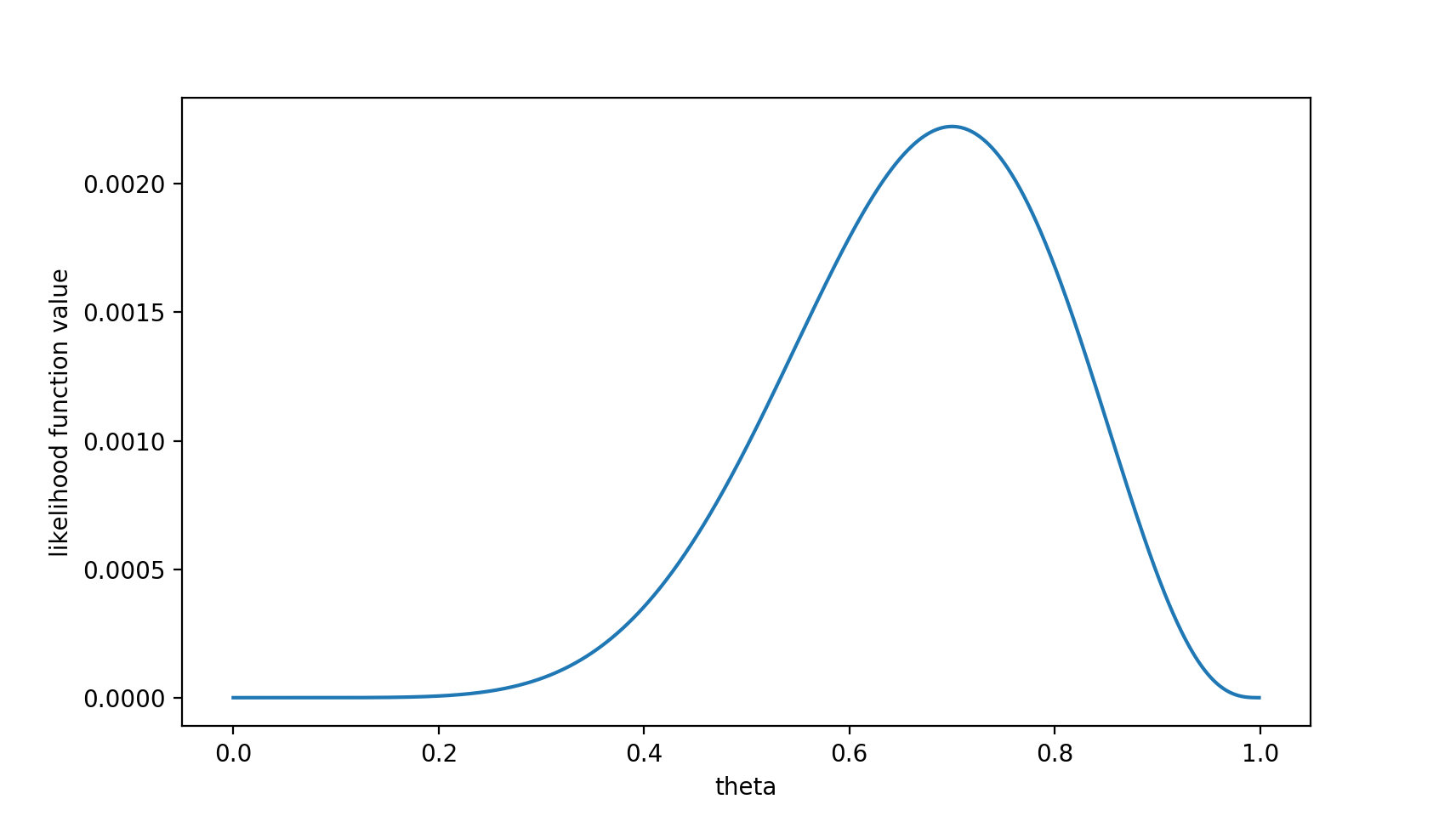可以看出，在θ=0.7\theta = 0.7时，似然函数取得最大值。
这样，我们已经完成了对θ\theta的最大似然估计。即，抛10次硬币，发现7次硬币正面向上，最大似然估计认为正面向上的概率是0.7。（ummm…这非常直观合理，对吧？）
且慢，一些人可能会说，硬币一般都是均匀的啊！ 就算你做实验发现结果是“反正正正正反正正正反”，我也不信θ=0.7\theta = 0.7。
这里就包含了贝叶斯学派的思想了——要考虑先验概率。 为此，引入了最大后验概率估计。
最大后验概率估计
最大似然估计是求参数θ\theta, 使似然函数$P(x_0 | \theta) 最大。最大后验概率估计则是想求最大。最大后验概率估计则是想求\theta使使P(x_0 | \theta) P(\theta)最大。求得的最大。求得的\theta不单单让似然函数大，不单单让似然函数大，\theta$自己出现的先验概率也得大。 （这有点像正则化里加惩罚项的思想，不过正则化里是利用加法，而MAP里是利用乘法）
MAP其实是在最大化P(θ∣x0)=P(x0∣θ)P(θ)P(x0)P(\theta|x_0) = \frac{P(x_0|\theta)P(\theta)}{P(x_0)}，不过因为x0x_0是确定的（即投出的“反正正正正反正正正反”），P(x0)P(x_0)是一个已知值，所以去掉了分母P(x0)P(x_0)（假设“投10次硬币”是一次实验，实验做了1000次，“反正正正正反正正正反”出现了n次，则P(x0)=n/1000P(x_0) = n/1000。总之，这是一个可以由数据集得到的值）。最大化P(θ∣x0)P(\theta | x_0)的意义也很明确，x0x_0已经出现了，要求θ\theta取什么值使P(θ∣x0)P(\theta | x_0)最大。顺带一提，P(θ∣x0)P(\theta | x_0)即后验概率，这就是“最大后验概率估计”名字的由来。
对于投硬币的例子来看，我们认为（”先验地知道“）θ\theta取0.5的概率很大，取其他值的概率小一些。我们用一个高斯分布来具体描述我们掌握的这个先验知识，例如假设P(θ)P(\theta)为均值0.5，方差0.1的高斯函数，如下图：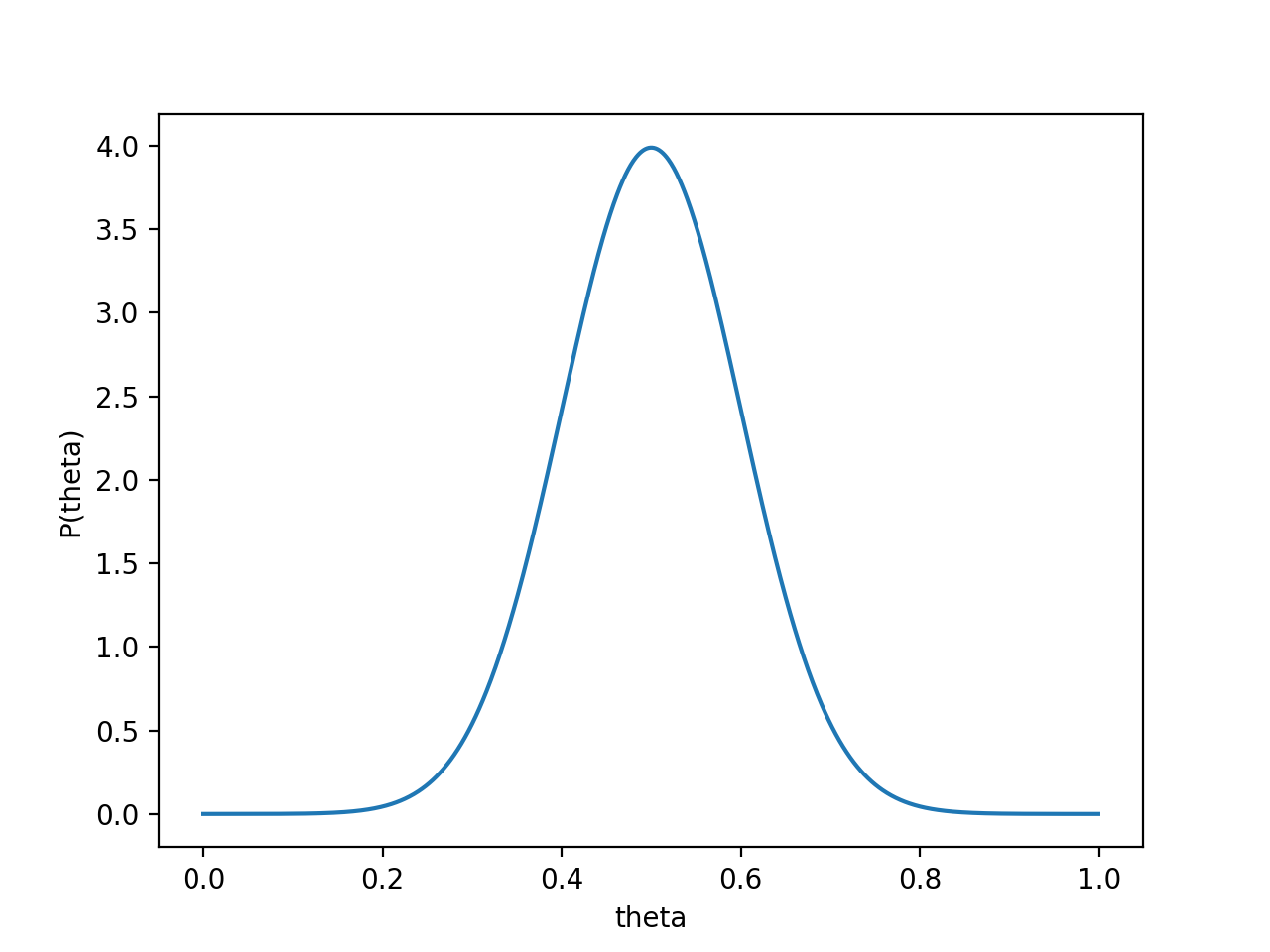则P(x0∣θ)P(θ)P(x_0 | \theta) P(\theta)的函数图像为：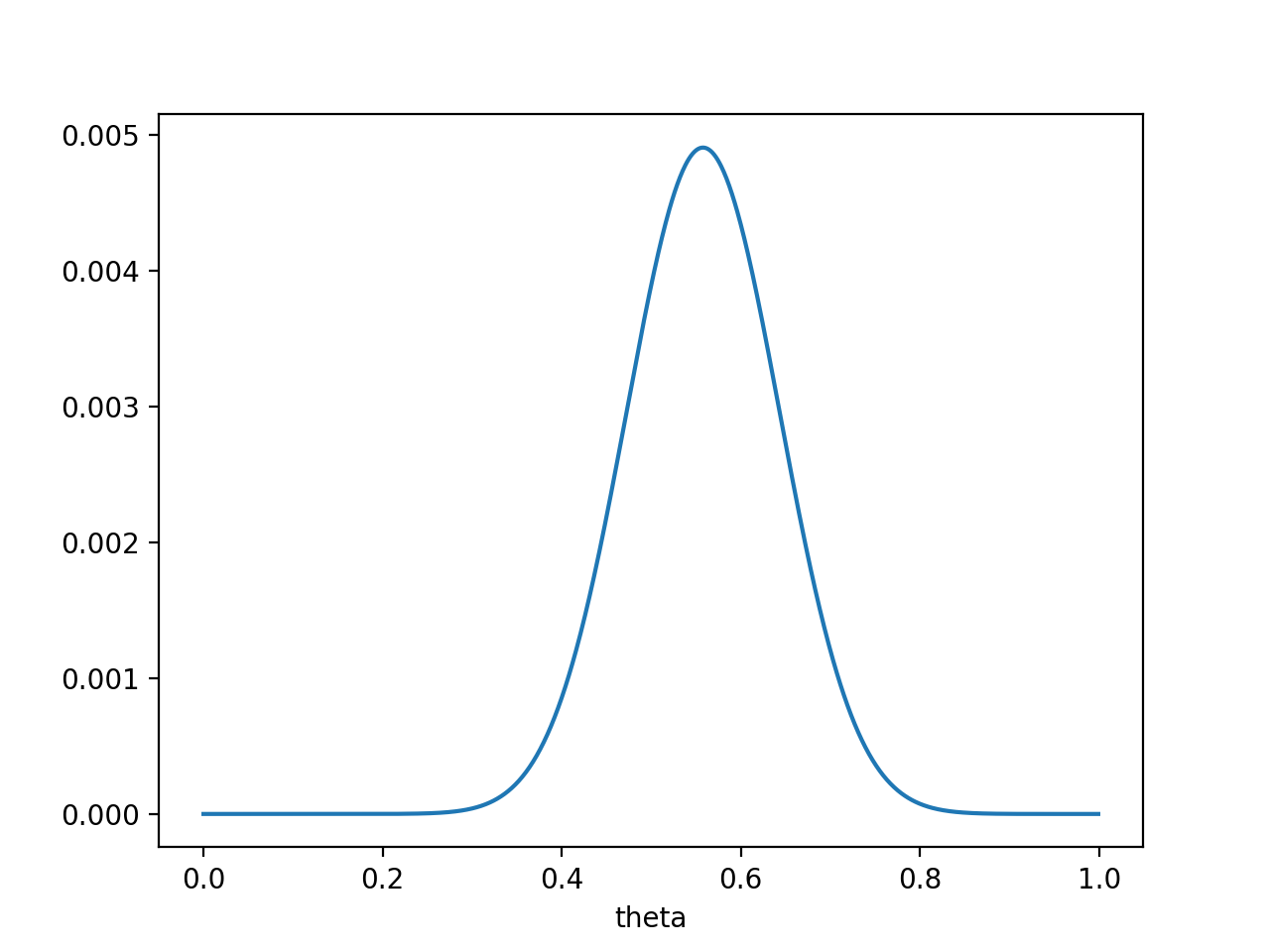注意，此时函数取最大值时，θ\theta取值已向左偏移，不再是0.7。实际上，在θ=0.558\theta = 0.558时函数取得了最大值。即，用最大后验概率估计，得到θ=0.558\theta = 0.558
最后，那要怎样才能说服一个贝叶斯派相信θ=0.7\theta = 0.7呢？你得多做点实验。。
如果做了1000次实验，其中700次都是正面向上，这时似然函数为: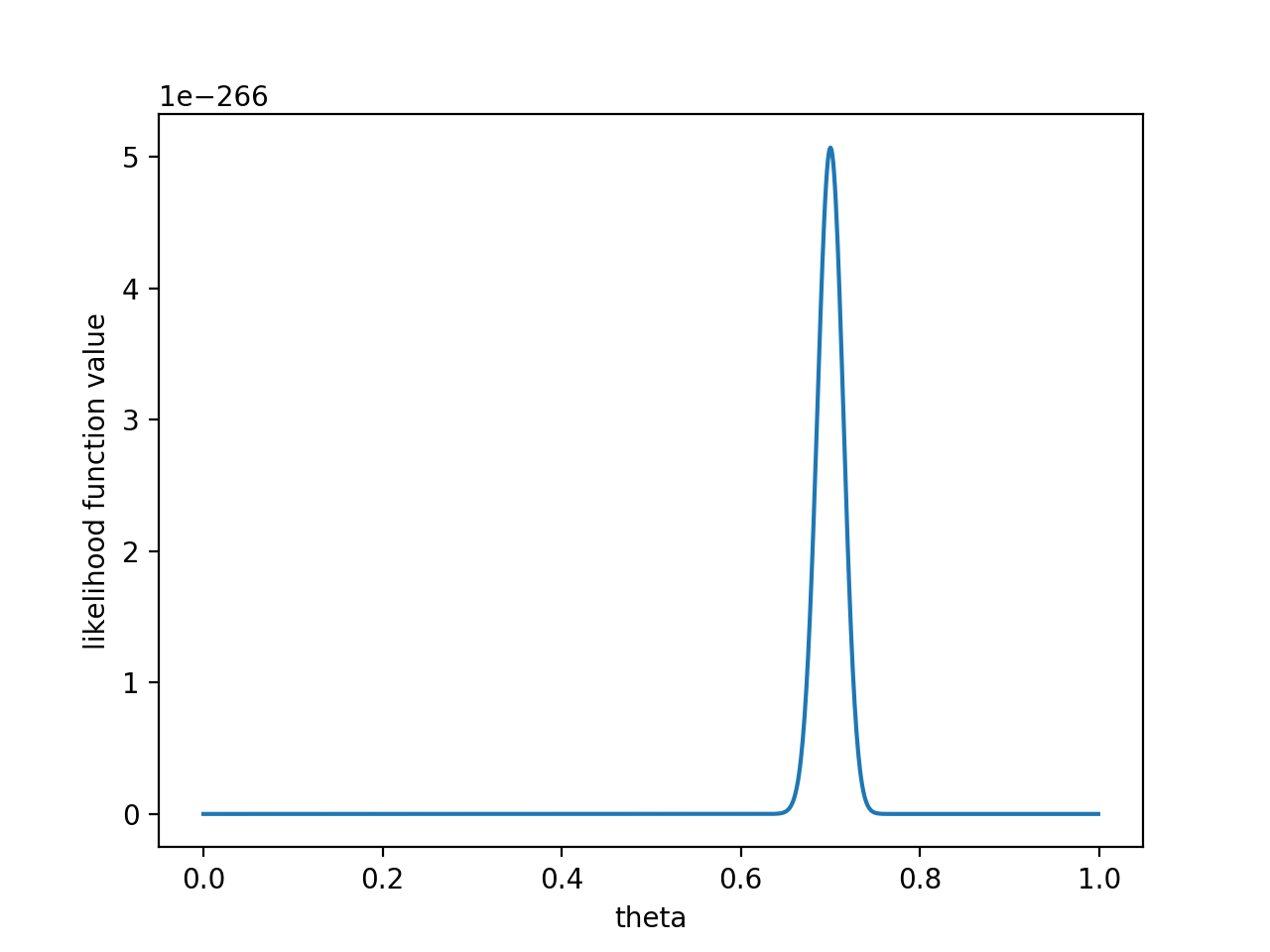如果仍然假设P(θ)P(\theta)为均值0.5，方差0.1的高斯函数，P(x0∣θ)P(θ)P(x_0 | \theta) P(\theta)的函数图像为：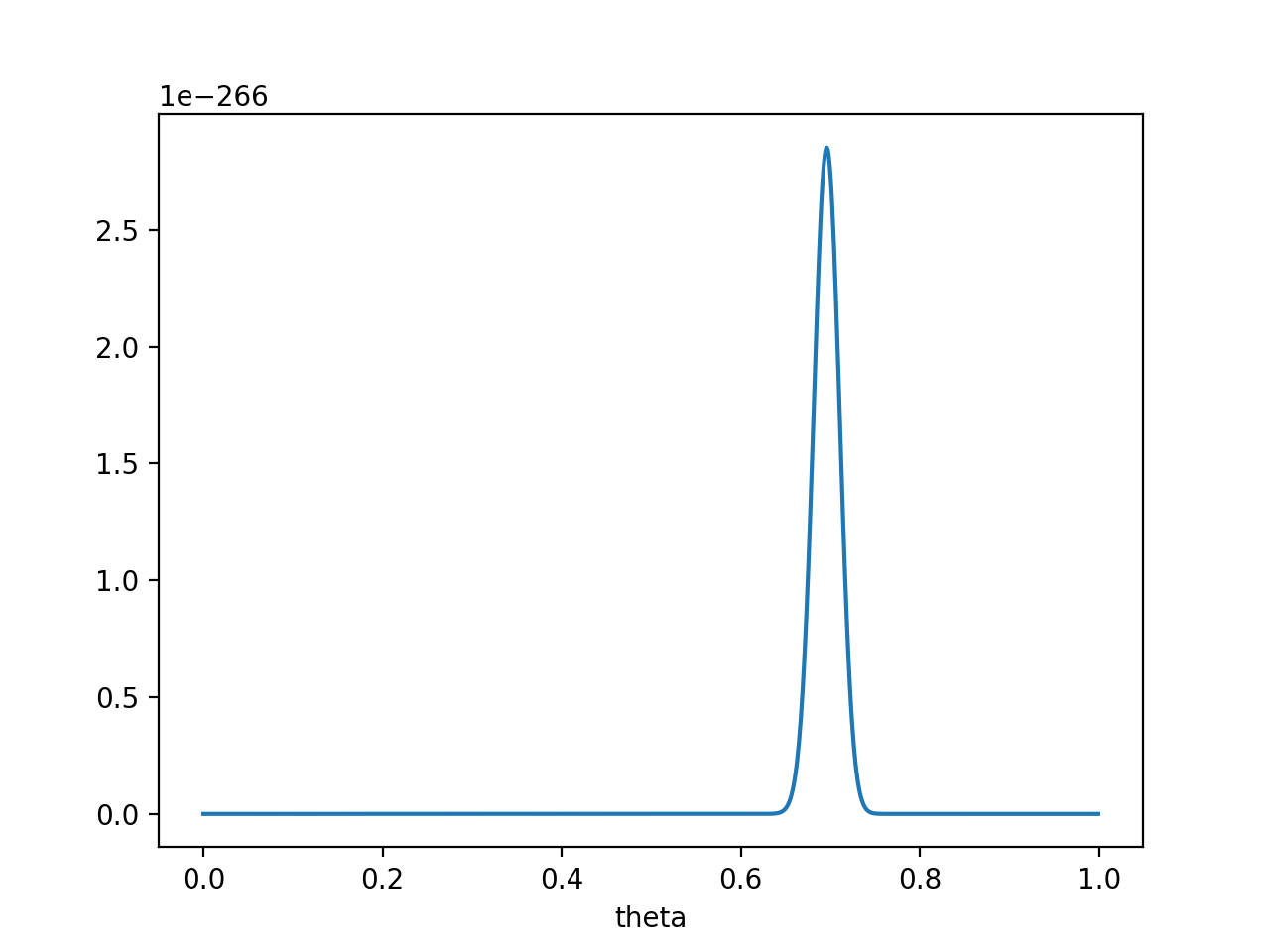在θ=0.696\theta = 0.696处，P(x0∣θ)P(θ)P(x_0 | \theta) P(\theta)取得最大值。
这样，就算一个考虑了先验概率的贝叶斯派，也不得不承认得把θ\theta估计在0.7附近了。
PS. 要是遇上了顽固的贝叶斯派，认为P(θ=0.5)=1P(\theta = 0.5) = 1 ，那就没得玩了。。 无论怎么做实验，使用MAP估计出来都是θ=0.5\theta = 0.5。这也说明，一个合理的先验概率假设是很重要的。（通常，先验概率能从数据中直接分析得到）
最大似然估计和最大后验概率估计的区别
相信读完上文，MLE和MAP的区别应该是很清楚的了。MAP就是多个作为因子的先验概率P(θ)P(\theta)。或者，也可以反过来，认为MLE是把先验概率P(θ)P(\theta)认为等于1，即认为θ\theta是均匀分布。
如果有说错的或者没说清楚的地方，欢迎留言指教！如果您更好的见解，也欢迎留言交流！
谢谢阅读！
作者: nebulaf91


展开全文机器学习 贝叶斯
• 最大似然估计似然函数最大似然估计离散型随机变量的最大似然估计连续型随机变量的最大似然估计数学期望离散型连续型参考 似然函数 最大似然估计 离散型随机变量的最大似然估计 连续型随机变量的最大似然估计 数学...
最大似然估计似然函数最大似然估计离散型随机变量的最大似然估计连续型随机变量的最大似然估计数学期望离散型连续型最大似然估计 交叉熵  KL散度最大似然估计与交叉熵的关系KL散度与交叉熵KL散度与JS散度KL散度JS散度参考
似然函数最大似然估计离散型随机变量的最大似然估计连续型随机变量的最大似然估计数学期望
随机变量包括离散型和连续型，数学期望的计算也分离散型和连续型。
离散型连续型最小化KL散度等价于最大化似然函数最大似然估计 交叉熵  KL散度
最大似然估计与交叉熵的关系KL散度与交叉熵KL散度与JS散度
KL散度JS散度参考
知乎最大似然估计
深度学习中交叉熵和KL散度和最大似然估计之间的关系


展开全文...